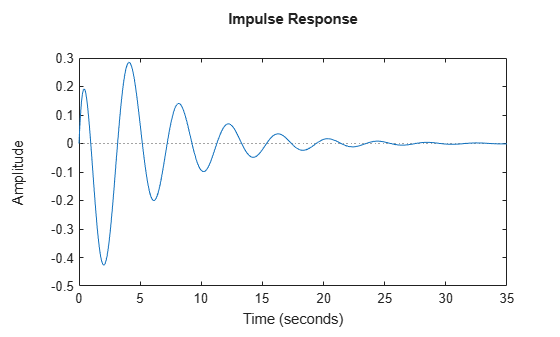Main Content

# lsiminfo

Compute linear response characteristics

## Syntax

```S = lsiminfo(y,t,yfinal) S = lsiminfo(y,t) S = lsiminfo(...,'SettlingTimeThreshold',ST) ```

## Description

`S = lsiminfo(y,t,yfinal)` takes the response data `(t,y) `and a steady-state value `yfinal` and returns a structure `S` containing the following performance indicators:

• `SettlingTime` — Settling time

• `Min` — Minimum value of Y

• `MinTime` — Time at which the minimum value is reached

• `Max` — Maximum value of Y

• `MaxTime` — Time at which the maximum value is reached

For SISO responses, `t` and `y` are vectors with the same length NS. For responses with NY outputs, you can specify `y` as an NS-by-NY array and `yfinal` as a NY-by-1 array. `lsiminfo` then returns an NY-by-1 structure array `S` of performance metrics for each output channel.

`S = lsiminfo(y,t)` uses the last sample value of `y` as steady-state value `yfinal`. ```s = lsiminfo(y)``` assumes `t = 1:NS`.

`S = lsiminfo(...,'SettlingTimeThreshold',ST)` lets you specify the threshold `ST` used in the settling time calculation. The response has settled when the error ```|y(t) - yfinal|``` becomes smaller than a fraction `ST` of its peak value. The default value is `ST=0.02` (2%).

## Examples

collapse all

Create the following continuous-time transfer function:

`$H\left(s\right)=\frac{s-1}{{s}^{3}+2{s}^{2}+3s+4}$`

`sys = tf([1 -1],[1 2 3 4]);`

Calculate the impulse response.

`[y,t] = impulse(sys);`

`impulse` returns the output response `y` and the time vector `t` used for simulation.

Compute the response characteristics using a final response value of `0`.

`s = lsiminfo(y,t,0)`
```s = struct with fields: SettlingTime: 22.8700 Min: -0.4268 MinTime: 2.0088 Max: 0.2847 MaxTime: 4.0733 ```

You can plot the impulse response and verify these response characteristics. For example, the time at which the minimum response value (`MinTime`) is reached is approximately 2 seconds.

`impulse(sys)`## See Also

Introduced in R2006a

## Support

#### Learn how to automatically tune PID controller gains

Download code examples